Want Some cool tricks for solving math problems in just second. Here it is.
If you always hated maths just because you were not able to understand some formulas, we got something for you. Here we got you some useful math’s tips you probably never learned in school and we hope now you would be able to solve problems with ease. Check some out here.Multiplying large numbers in your head

### Learning the nine times tableLearning the nine times table

### The butterfly method for adding and subtracting fractionsThe butterfly method for adding and subtracting fractions

### Multiplying by 11 (example: 11×32)Multiplying by 11 (example: 11×32)

### Memorizing PiMemorizing Pi

### Multiplying by 6, 7, 8, and 9 using only your handsMultiplying by 6, 7, 8, and 9 using only your hands

### Finding the percentage of a numberFinding the percentage of a number

### Finding a fraction of a whole numberFinding a fraction of a whole number

### Converting Celcius to Fahrenheit and vice versaConverting Celcius to Fahrenheit and vice versaMath Tricks

### 10. Last

Eating chocolate while studying math can improve your math skills

## Math Tricks Android App

This App is designed for those who want to learn interesting mathematical tricks to speed up the calculating. These tricks will help solve part of the mathematical problems and tasks much faster than classical.
When you learn these mathematical tricks, you’ll be able to show off your skills to friends and prove to them that you have a talent for mathematics. New skills you can use in the store, at school, at college, at work – wherever thanks to quick calculation skills can save a lot of precious time.
Developer: Antoni
Price: Free
••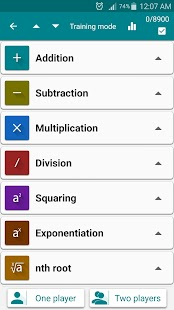•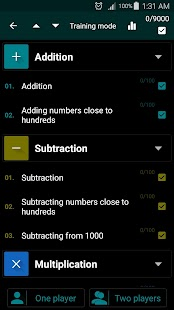•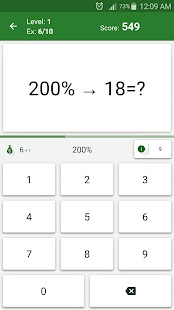•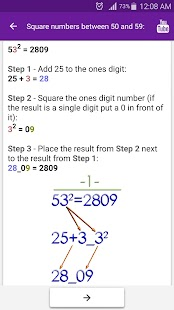•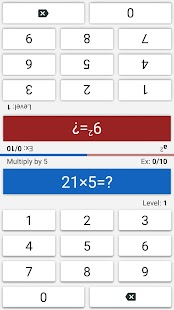•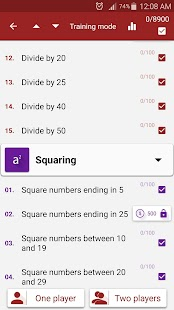•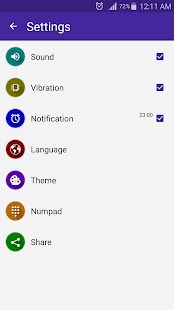•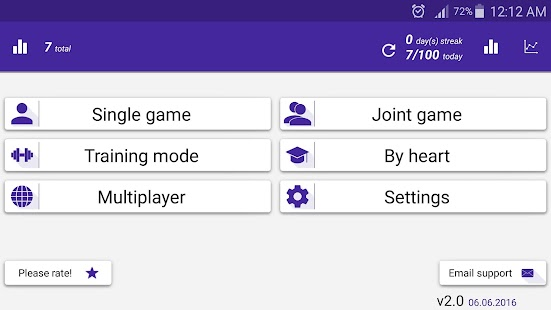•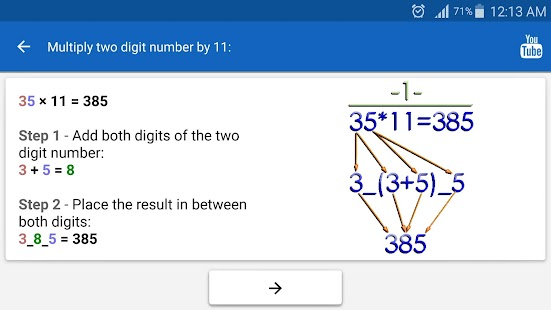•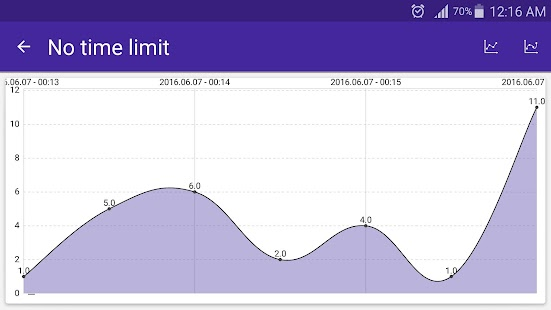•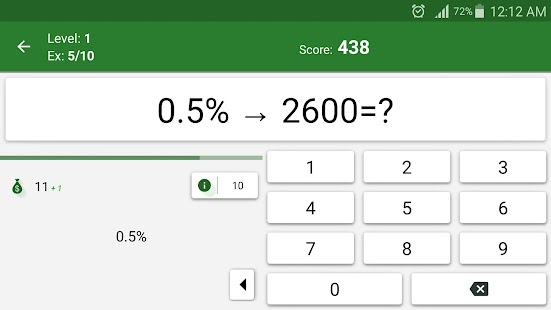Also, See this Video for more Math Tricks.